## MP Board Class 6th Maths Solutions Chapter 8 Decimals Ex 8.1

Question 1.
Write the following as numbers in the given table.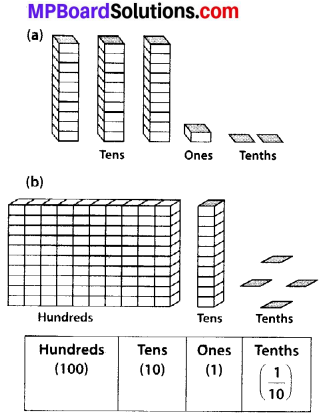Solution: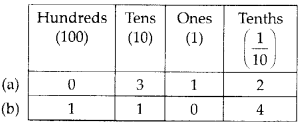Question 2.
Write the following decimals in the place value table.
(a) 19.4
(b) 0.3
(c) 10.6
(d) 205.9
Solution: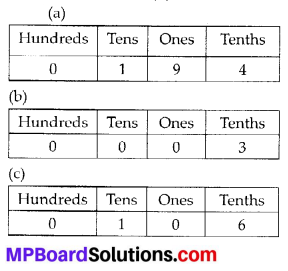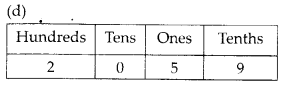Question 3.
Write each of the following as decimals:
(a) Seven-tenths
(b) Two tens and nine-tenths
(c) Fourteen point six
(d) One hundred and two ones
(e) Six hundred point eight
Solution:
(a) Seven-tenths = 7 tenths = $$\frac{7}{10}$$ = 0.7
(b) Two tens and nine-tenths = $$2 \times 10+\frac{9}{10}$$
= 20 + 0.9 = 20.9
(c) Fourteen point six = 14.6
(d) One hundred and two-ones = 100 + 2 × 1
= 100 + 2 = 102
(e) Six hundred point eight = 600.8

Question 4.
Write each of the following as decimals: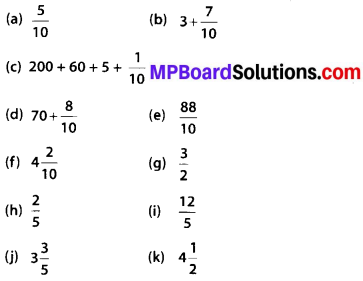Solution: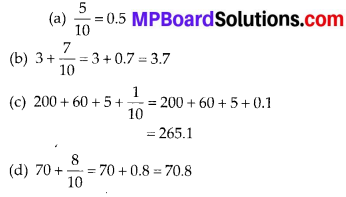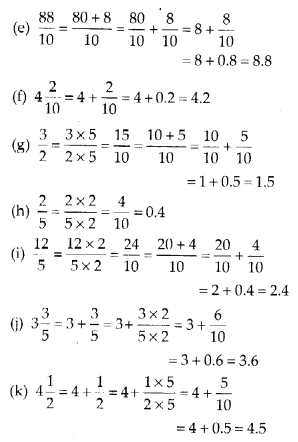Question 5.
Write the following decimals as fractions. Reduce the fractions to lowest form.
(a) 0.6
(b) 2.5
(c) 1.0
(d) 3.8
(e) 13.7
(f) 21.2
(g) 6.4
Solution: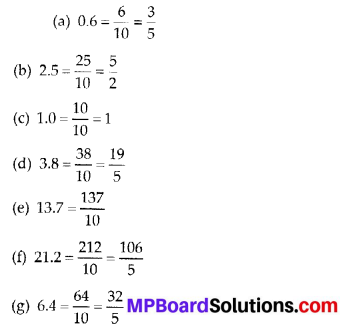Question 6.
Express the following as cm using decimals.
(a) 2 mm
(b) 30 mm
(c) 116 mm
(d) 4 cm 2 mm
(e) 162 mm
(f) 83 mm
Solution: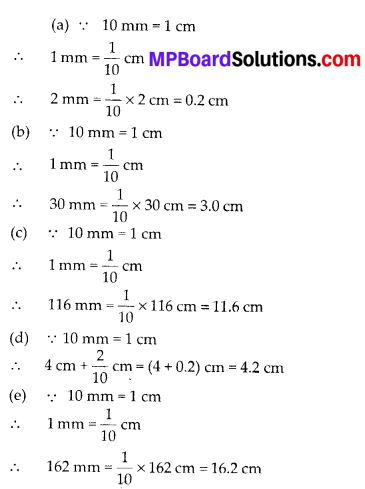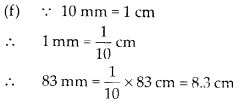Question 7.
Between which two whole numbers on the number line are the given numbers lie?
Which of these whole numbers is nearer the number?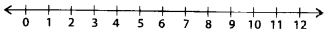Solution:
(a) 0.8
(b) 5.1
(C) 2.6
(d) 6.4
(e) 9.1
(f) 4.9
Solution:
(a) 0.8 lies between 0 and 1. From 0 to 1, 0.8 is nearer to 1
(b) 5.1 lies between 5 and 6. From 5 to 6, 5.1 is nearer to 5.
(c) 2.6 lies between 2 and 3. From 2 to 3, 2.6 is nearer to 3.
(d) 6.4 lies between 6 and 7. From 6 to 7, 6.4 is nearer to 6.
(e) 9.1 lies between 9 and 10. From 9 to 10, 9.1 is nearer to 9.
(f) 4.9 lies between 4 and 5. From 4 to 5, 4.9 is nearer to 5.Question 8.
Show the following numbers on the number line.
(a) 0.2
(b) 1.9
(c) 1.1
(d) 2.5
Solution: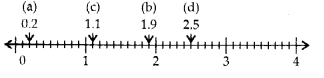Question 9.
Write the decimal number represented by the points A, B, C, D on the given number line.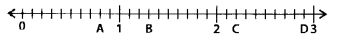Solution: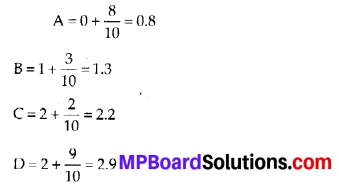Question 10.
(a) The length of Ramesh’s notebook is 9 cm 5 mm. What will be its length in cm?
(b) The length of a young gram plant is 65 mm. Express its length in cm.
Solution:
(a) 9 cm 5 mm = 9 cm + 5 mm
= $$\left(9+\frac{5}{10}\right)$$ cm = 9.5 cm

(b) 65 mm = $$\frac{65}{10}$$ cm = 6.5 cm